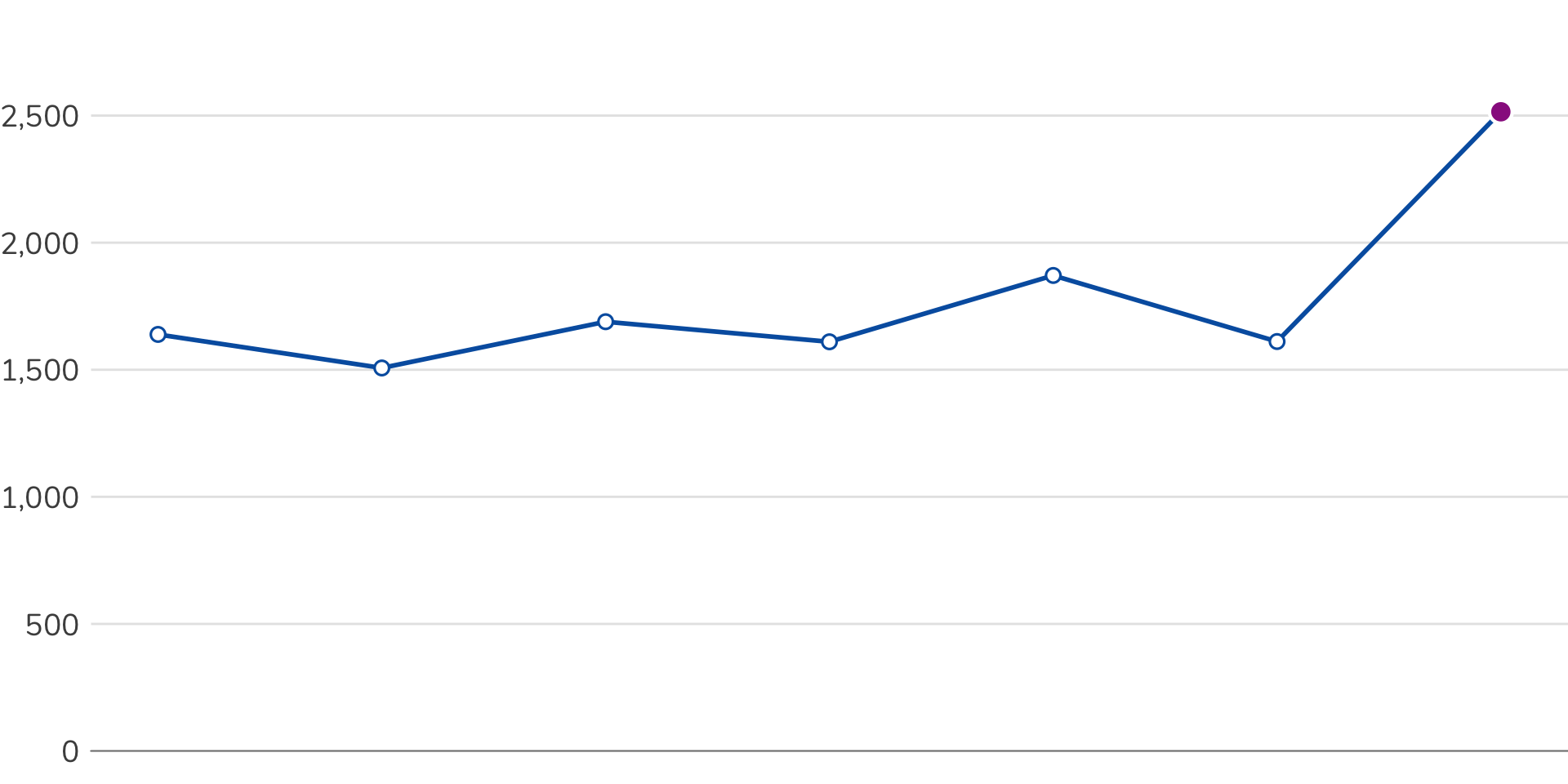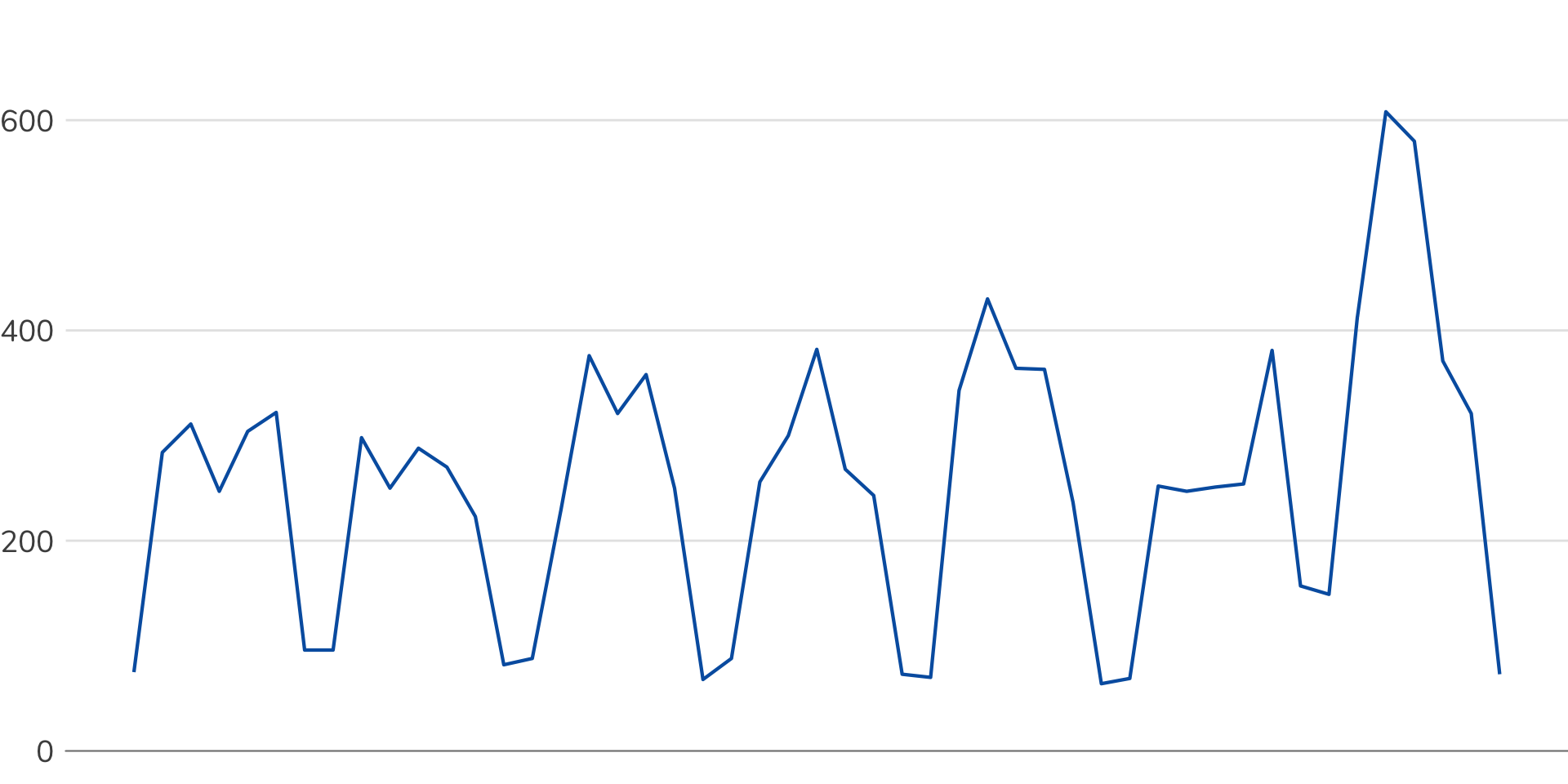### General Note

This example is part of a larger set of examples of using Google Analytics with R:

### Overview

This example is an exploration of using Holt-Winters forecasting for anomaly detection. Mainly, it’s geared towards an explanation of time-series decomposition and how that technique can be used to identify whether some set of data falls outside of an expected range.

### Setup/Config

``````# Load the necessary libraries.
if (!require("pacman")) install.packages("pacman")``````
``## Loading required package: pacman``
``````pacman::p_load(googleAnalyticsR,  # How we actually get the Google Analytics data
tidyverse,         # Includes dplyr, ggplot2, and others; very key!
splitstackshape,   # For some data munging (probably better to use a tidyverse package)
plotly,            # We're going to make the charts interactive
scales,            # Useful for some number formatting in the visualizations
lubridate,         # For the wday function
tools)             # For our stepwise regression

# Authorize GA. Depending on if you've done this already and a .ga-httr-oauth file has
# been saved or not, this may pop you over to a browser to authenticate.
ga_auth(token = ".httr-oauth", new_user = TRUE)``````
``## Token cache file: .httr-oauth``
``````# Set the total number of weeks to be used. The most recent week will be the "checked" week,
# while the weeks before will be used to build the forecast.
total_weeks <- 7

# Set the view ID and the date range. If you want to, you can swap out the Sys.getenv()
# call and just replace that with a hardcoded value for the view ID.
view_id <- Sys.getenv("GA_VIEW_ID")
end_date <- Sys.Date() - wday(Sys.Date())     # The most recent Saturday
start_date <- end_date - total_weeks * 7 + 1  # Start date based on total_weeks

# Define our theme -- this will be used later for visualizations of the full-sized charts
theme_hw <- theme_bw() +
theme(text = element_text(family="Nunito"),
plot.title = element_text(size = 10, face = "bold", hjust = 0.5),
plot.margin = margin(1.5,0,0,0,"cm"),
axis.text.x = element_blank(),
axis.text.y = element_text(size = 14),
axis.title = element_blank(),
axis.title.x = element_text(size = 10, hjust = 0.5),
axis.ticks = element_blank(),
axis.line.x = element_line(color = "gray50"),
axis.line.y = element_blank(),
legend.title = element_blank(),
legend.background = element_blank(),
legend.position = "top",
legend.justification = "center",
panel.border = element_blank(),
panel.grid.major.x = element_blank(),
panel.grid.major.y = element_line(size=0.5, colour = "gray90"),
panel.grid.minor = element_blank())

# And, a theme for the time-series decomposition
theme_sparklines <- theme_bw() +
theme(axis.text = element_blank(),
axis.title = element_blank(),
axis.ticks = element_blank(),
axis.line = element_blank(),
legend.title = element_blank(),
legend.background = element_blank(),
legend.position = "none",
legend.justification = "center",
strip.text.x = element_text(face = "bold", size = 14, colour = "grey10", family="Nunito"),
strip.text.y = element_text(face = "bold", size = 14, colour = "grey10",
angle = 180, hjust=1, family="Nunito"),
strip.background = element_blank(),
panel.border = element_blank(),
panel.grid.major = element_blank(),
panel.grid.minor = element_blank(),
panel.spacing = unit(0,"in"),
panel.background = element_rect(fill = NA, color = NA))``````

### Pull the Data

This is a simple data pull. We’re just pulling data by day (because each day is a different day of the week) and sessions. And, since Google Analytics has a “Day of Week Name” dimension, we’ll go ahead and pull that, too (although we could have easily calculated this afterwards).

``````# Pull the data. See ?google_analytics_4() for additional parameters. The anti_sample = TRUE
# parameter will slow the query down a smidge and isn't strictly necessary, but it will
# ensure you do not get sampled data.
date_range = c(start_date, end_date),
metrics = "sessions",
dimensions = "date",
anti_sample = TRUE)``````

### Plot the Data as Weekly Data

We pulled the data daily, so we’re going to roll this up to be weekly and then plot it to show how the data might look in a dashboard that includes a weekly sparkline.

``````# Aggregate the data to be weekly
ga_data_weekly <- ga_data %>%
mutate(week = date - wday(date) + 1) %>%
group_by(week) %>%
summarise(sessions = sum(sessions)) %>%
mutate(last_week_sessions = ifelse(week == max(week), sessions, NA ))

# Build a plot
ga_plot_weekly <- ggplot(ga_data_weekly, mapping = aes(x = week, y = sessions)) +
geom_line(color = "#0060AF", size = 1) +
geom_point(aes(y=sessions), size=3, shape = 19, colour = "#0060AF") +
geom_point(aes(y=sessions), size=3, shape = 20, colour = "white") +
geom_point(aes(y=last_week_sessions), size=5, colour = "white") +
geom_point(aes(y=last_week_sessions), size=3.5, colour = "#9A258F") +
scale_y_continuous(label=comma, expand = c(0, 0), limits = c(0, max(ga_data_weekly\$sessions) * 1.03)) +
labs(x = " ") +
theme_hw

# Plot the data
ga_plot_weekly``````### Data Munging

A lot of this is for the purposes of the visualization, but, essentially, we need to break the data into the “data before last week” (this is the data we’ll use to build forecast) and the “data to be evaluated” (the data from last week).

``````# Determine how many rows of the data will be used to build the forecast. This
# is everything except the last week.
rowcount_forecast <- nrow(ga_data) - 7

# Also figure out the date where the cutoff is between training and forecast
cutoff_date <- ga_data[rowcount_forecast,1]

# We actually want to shift this over a little bit to fall between two points when we plot
cutoff_date <- (2*as.numeric(cutoff_date) + 1)/2

# Make a data set that removes the "rows to be evaluated." This will get
# used both to generate the time series for the forecast as well as for modeling
ga_data_training <- ga_data %>%
top_n(-rowcount_forecast, wt = date)

# Get the date values for the forecast period
dates_forecast <- ga_data %>%
top_n(7, wt = date) %>%
dplyr::select(date)

# Make a time-series object using the data for the training period. This
# is what we'll use to build the forecast
ga_data_ts <- ga_data_training[] %>%
ts(frequency = 7)

# Start building out our master data for plotting by adding a column that
# has just the data being used for the training
ga_data_plot <- ga_data %>%
left_join(ga_data_training, by = c(date = "date"))

# Rename columns to be a bit clearer
names(ga_data_plot) <- c("date", "sessions_all", "sessions_training")

# Add a column that is just the actuals data of interest
ga_data_plot <- ga_data_plot %>%
mutate(sessions_assess = ifelse(is.na(sessions_training), sessions_all, NA))``````

### Visualizing the Data

The basic view of the raw data:We’re going to split this data up into two different groups: